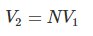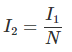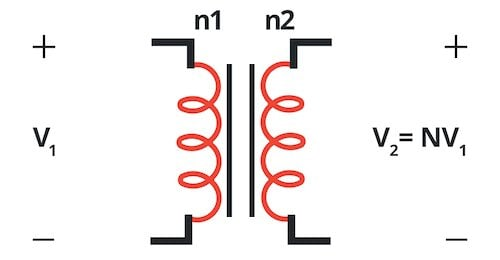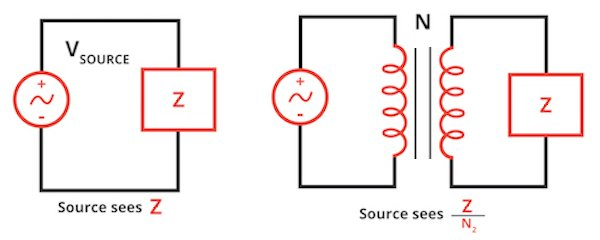# Transformers

Chapter 5 - Impedance Matching and Power Transfer

AC electrical systems make extensive use of transformers. A transformer is equivalent to two inductors arranged in such a way as to enhance magnetic coupling. The strong magnetic coupling between these two inductors allows electrical energy to be transferred from one to the other without a physical conductive connection. In other words, normal electric current cannot flow from one side of the transformer to the other, but by efficiently transferring electrical energy by means of a time-varying magnetic field, the device acts as though it allows alternating current to flow.Figure 1. A transformer

The two inductors in a transformer are called windings. The primary winding receives the input signal, and the secondary winding generates the output signal. As shown in Figure 1, when we draw an inductor, the primary winding is on the left and the secondary winding is on the right. The vertical lines in the symbol represent the use of a core material; coiling a wire around a magnetic core (instead of air) results in a stronger magnetic field.

## The Turns Ratio

As indicated above, a transformer provides electrical isolation between two portions of a system: the absence of a physical conductive connection means that the transformer will block DC voltages and currents. Isolation is an important feature in certain situations, but it turns out that transformers provide additional functionality that is an essential aspect of electric power distribution: they can “transform” AC voltage and current amplitudes.

Voltage and current transformation are achieved by constructing a transformer with windings that have an unequal number of “turns”—one turn corresponds to winding the wire around the core one time. More specifically, the change in voltage or current amplitude is determined by the ratio of the number of turns in the secondary winding to the number of turns in the primary winding. If the number of secondary turns is denoted by n2 and the number of primary turns is denoted by n1, the turns ratio (N) is calculated as follows:If we know the voltage across the primary winding (V1) and the current flowing through the primary winding (I1), all we need is the turns ratio in order to calculate the voltage generated by the secondary winding (V2) and the current flowing through the secondary winding (I2):Figure 2. The turns ratio establishes the relationship between the transformer’s input and output voltage.

Note that N can be greater than or less than one—i.e., a transformer can be used to create a secondary voltage that is higher or lower than the primary voltage.

## Types of Transformers

A transformer that is designed to generate an output voltage that is higher than the input voltage is called a step-up transformer. An important application of step-up transformers is greatly increasing the voltage generated by a power plant so that the electrical energy can be efficiently transferred over long distances.

A transformer that generates an output voltage that is lower than the input voltage is a step-down transformer. The power grid uses step-down transformers to reduce the extremely high transmission voltage to amplitudes that are appropriate for industrial and household use.

If a transformer has a turns ratio of 1, it is called an isolation transformer because there is no change in voltage amplitude and thus it provides only electrical isolation.

A center-tapped transformer has a terminal connected to the center of the secondary winding. This allows a single transformer to generate two output voltages that have half of the amplitude that appears across the entire secondary winding. Residential electrical systems in the United States use a center-tapped transformer to provide both 115 VRMS for lower-power devices and 230 VRMS for high-power appliances such as air conditioners and water heaters.Figure 3. An example of how a center-tapped transformer can be used.

## Transforming Impedance

We’ll conclude this page with a discussion of an interesting way in which a transformer influences circuit behavior. The effect is called impedance reflection.

If a voltage source is connected to a load, the impedance “seen by” the source is simply the impedance of the load. This use of the verb “to see” is an attempt to concisely convey the technique of analyzing a subcircuit from the perspective of a separate subcircuit. In the case of a source and a resistive load, for example, the load component has a physical value, say 100 Ω, but the effect of the component as experienced by the source might correspond to a different resistance.Figure 4. Impedance reflection

The presence of a transformer can alter the impedance seen by a voltage source. This is not too surprising when we consider that impedance is related to voltage and current and that a transformer with N ≠ 1 alters the voltage across and current through a load component on the secondary side. It turns out that a voltage source on the primary side of a transformer sees an impedance of Z divided by N2 on the secondary side, where Z is the physical impedance of the load component and N is the turns ratio.

## Transformers Review

We’ve discussed some basic information related to transformers. This page also introduced the phenomenon of transformer impedance reflection, but it didn’t explain the significance of impedance reflection in the context of circuit design. We’ll explore that issue in the next page, which presents the concept of maximum power transfer.